## Free Energy

Free energy, called Gibbs free energy (G), is usable energy or energy that is available to do work.

### Learning Objectives

Discuss the concept of free energy.

### Key Takeaways

#### Key Points

• Every chemical reaction involves a change in free energy, called delta G (∆G).
• To calculate ∆G, subtract the amount of energy lost to entropy (∆S) from the total energy change of the system; this total energy change in the system is called enthalpy (∆H ): ΔG=ΔH−TΔS.
• Endergonic reactions require an input of energy; the ∆G for that reaction will be a positive value.
• Exergonic reactions release free energy; the ∆G for that reaction will be a negative value.

#### Key Terms

• exergonic reaction: A chemical reaction where the change in the Gibbs free energy is negative, indicating a spontaneous reaction
• endergonic reaction: A chemical reaction in which the standard change in free energy is positive, and energy is absorbed
• Gibbs free energy: The difference between the enthalpy of a system and the product of its entropy and absolute temperature

### Free Energy

Since chemical reactions release energy when energy-storing bonds are broken, how is the energy associated with chemical reactions quantified and expressed? How can the energy released from one reaction be compared to that of another reaction?

A measurement of free energy is used to quantitate these energy transfers. Free energy is called Gibbs free energy (G) after Josiah Willard Gibbs, the scientist who developed the measurement. Recall that according to the second law of thermodynamics, all energy transfers involve the loss of some amount of energy in an unusable form such as heat, resulting in entropy. Gibbs free energy specifically refers to the energy associated with a chemical reaction that is available after accounting for entropy. In other words, Gibbs free energy is usable energy or energy that is available to do work.

### Calculating ∆G

Every chemical reaction involves a change in free energy, called delta G (∆G). The change in free energy can be calculated for any system that undergoes a change, such as a chemical reaction. To calculate ∆G, subtract the amount of energy lost to entropy (denoted as ∆S) from the total energy change of the system. This total energy change in the system is called enthalpy and is denoted as ∆H. The formula for calculating ∆G is as follows, where the symbol T refers to absolute temperature in Kelvin (degrees Celsius + 273): G=ΔH−TΔS.

The standard free energy change of a chemical reaction is expressed as an amount of energy per mole of the reaction product (either in kilojoules or kilocalories, kJ/mol or kcal/mol; 1 kJ = 0.239 kcal) under standard pH, temperature, and pressure conditions. Standard pH, temperature, and pressure conditions are generally calculated at pH 7.0 in biological systems, 25 degrees Celsius, and 100 kilopascals (1 atm pressure), respectively. It is important to note that cellular conditions vary considerably from these standard conditions; therefore, standard calculated ∆G values for biological reactions will be different inside the cell.

### Endergonic and Exergonic Reactions

If energy is released during a chemical reaction, then the resulting value from the above equation will be a negative number. In other words, reactions that release energy have a ∆G < 0. A negative ∆G also means that the products of the reaction have less free energy than the reactants because they gave off some free energy during the reaction. Reactions that have a negative ∆G and, consequently, release free energy, are called exergonic reactions. Exergonic means energy is exiting the system. These reactions are also referred to as spontaneous reactions because they can occur without the addition of energy into the system. Understanding which chemical reactions are spontaneous and release free energy is extremely useful for biologists because these reactions can be harnessed to perform work inside the cell. An important distinction must be drawn between the term spontaneous and the idea of a chemical reaction that occurs immediately. Contrary to the everyday use of the term, a spontaneous reaction is not one that suddenly or quickly occurs. The rusting of iron is an example of a spontaneous reaction that occurs slowly, little by little, over time.

If a chemical reaction requires an input of energy rather than releasing energy, then the ∆G for that reaction will be a positive value. In this case, the products have more free energy than the reactants. Thus, the products of these reactions can be thought of as energy-storing molecules. These chemical reactions are called endergonic reactions; they are non-spontaneous. An endergonic reaction will not take place on its own without the addition of free energy.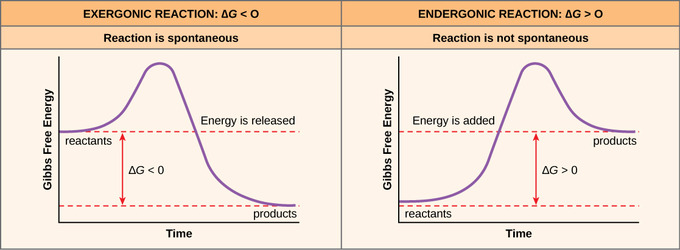Exergonic and Endergonic Reactions: Exergonic and endergonic reactions result in changes in Gibbs free energy. Exergonic reactions release energy; endergonic reactions require energy to proceed.

### Free Energy and Biological Processes

In a living cell, chemical reactions are constantly moving towards equilibrium, but never reach it. A living cell is an open system: materials pass in and out, the cell recycles the products of certain chemical reactions into other reactions, and chemical equilibrium is never reached. In this way, living organisms are in a constant energy-requiring, uphill battle against equilibrium and entropy.

When complex molecules, such as starches, are built from simpler molecules, such as sugars, the anabolic process requires energy. Therefore, the chemical reactions involved in anabolic processes are endergonic reactions. On the other hand, the catabolic process of breaking sugar down into simpler molecules releases energy in a series of exergonic reactions. As in the example of rust above, the breakdown of sugar involves spontaneous reactions, but these reactions don’t occur instantaneously. An important concept in the study of metabolism and energy is that of chemical equilibrium. Most chemical reactions are reversible. They can proceed in both directions, releasing energy into their environment in one direction, and absorbing it from the environment in the other direction.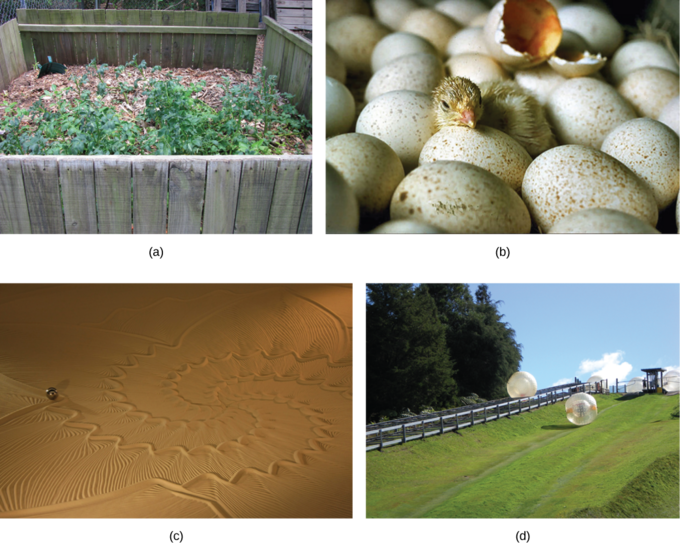Endergonic and Exergonic Processes: Shown are some examples of endergonic processes (ones that require energy) and exergonic processes (ones that release energy). These include (a) a compost pile decomposing, (b) a chick hatching from a fertilized egg, (c) sand art being destroyed, and (d) a ball rolling down a hill.

## The First Law of Thermodynamics

The first law of thermodynamics states that energy can be transferred or transformed, but cannot be created or destroyed.

### Learning Objectives

Describe the first law of thermodynamics

### Key Takeaways

#### Key Points

• According to the first law of thermodynamics, the total amount of energy in the universe is constant.
• Energy can be transferred from place to place or transformed into different forms, but it cannot be created or destroyed.
• Living organisms have evolved to obtain energy from their surroundings in forms that they can transfer or transform into usable energy to do work.

#### Key Terms

• first law of thermodynamics: A version of the law of conservation of energy, specialized for thermodynamical systems, that states that the energy of an isolated system is constant and can neither be created nor destroyed.
• work: A measure of energy expended by moving an object, usually considered to be force times distance. No work is done if the object does not move.

Thermodynamics is the study of heat energy and other types of energy, such as work, and the various ways energy is transferred within chemical systems. “Thermo-” refers to heat, while “dynamics” refers to motion.

### The First Law of Thermodynamics

The first law of thermodynamics deals with the total amount of energy in the universe. The law states that this total amount of energy is constant. In other words, there has always been, and always will be, exactly the same amount of energy in the universe.

Energy exists in many different forms. According to the first law of thermodynamics, energy can be transferred from place to place or changed between different forms, but it cannot be created or destroyed. The transfers and transformations of energy take place around us all the time. For instance, light bulbs transform electrical energy into light energy, and gas stoves transform chemical energy from natural gas into heat energy. Plants perform one of the most biologically useful transformations of energy on Earth: they convert the energy of sunlight into the chemical energy stored within organic molecules.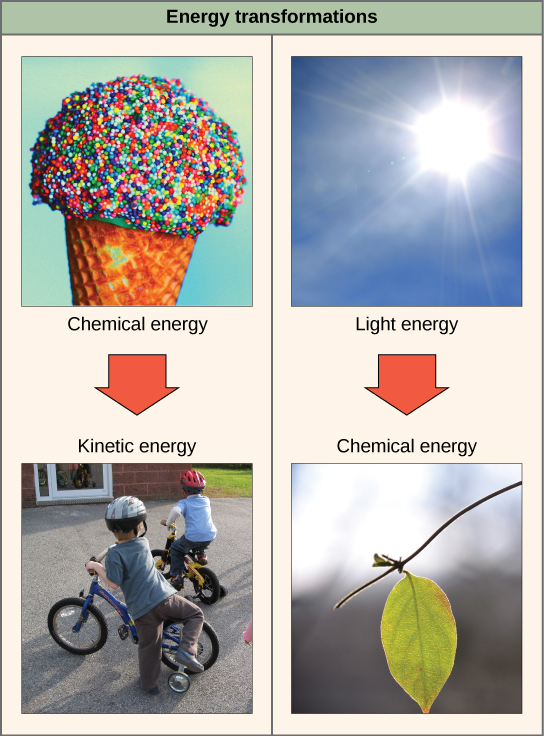The first law of thermodynamics: Shown are two examples of energy being transferred from one system to another and transformed from one form to another. Humans can convert the chemical energy in food, like this ice cream cone, into kinetic energy by riding a bicycle. Plants can convert electromagnetic radiation (light energy) from the sun into chemical energy.

### The System and Surroundings

Thermodynamics often divides the universe into two categories: the system and its surroundings. In chemistry, the system almost always refers to a given chemical reaction and the container in which it takes place. The first law of thermodynamics tells us that energy can neither be created nor destroyed, so we know that the energy that is absorbed in an endothermic chemical reaction must have been lost from the surroundings. Conversely, in an exothermic reaction, the heat that is released in the reaction is given off and absorbed by the surroundings. Stated mathematically, we have:

$\Delta \text{E}=\Delta \text{E}_{\text{sys}}+\Delta \text{E}_{\text{surr}}=0$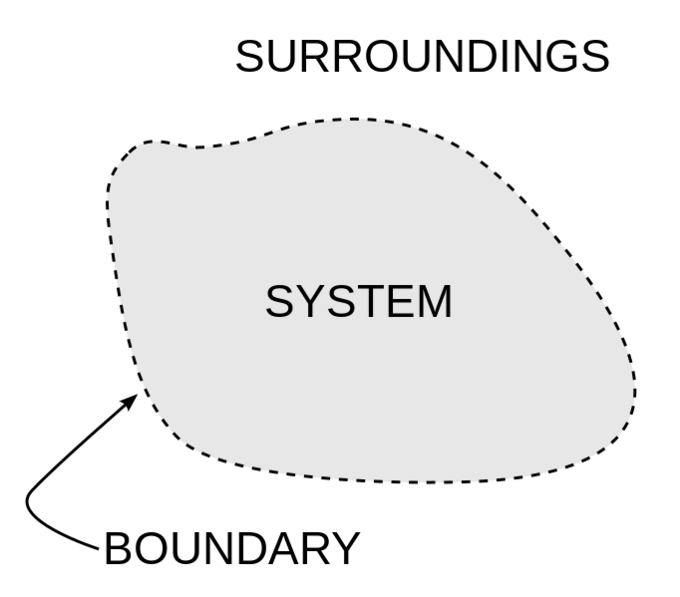The system and surroundings: A basic diagram showing the fundamental distinction between the system and its surroundings in thermodynamics.

### Heat and Work

We know that chemical systems can either absorb heat from their surroundings, if the reaction is endothermic, or release heat to their surroundings, if the reaction is exothermic. However, chemical reactions are often used to do work instead of just exchanging heat. For instance, when rocket fuel burns and causes a space shuttle to lift off from the ground, the chemical reaction, by propelling the rocket, is doing work by applying a force over a distance.

If you’ve ever witnessed a video of a space shuttle lifting off, the chemical reaction that occurs also releases tremendous amounts of heat and light. Another useful form of the first law of thermodynamics relates heat and work for the change in energy of the internal system:

$\Delta \text{E}_{\text{sys}}=\text{Q}+\text{W}$

While this formulation is more commonly used in physics, it is still important to know for chemistry.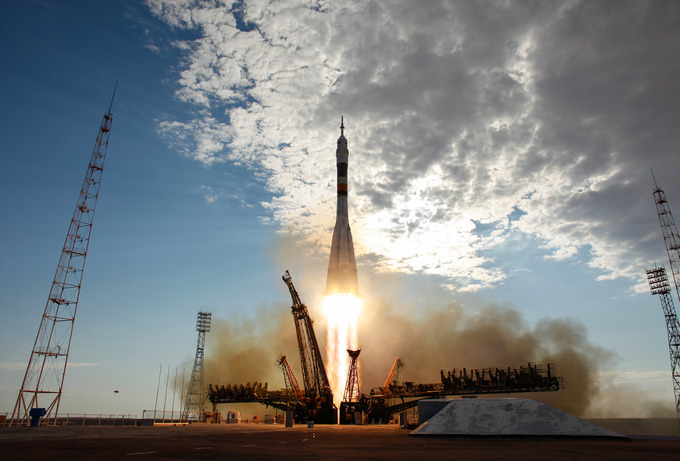Rocket launch: The powerful chemical reaction propelling the rocket lets off tremendous heat to the surroundings and does work on the surroundings (the rocket) as well.

## The Second Law of Thermodynamics

The second law of thermodynamics states that every energy transfer increases the entropy of the universe due to the loss of usable energy.

### Learning Objectives

Explain how living organisms can increase their order despite the second law of thermodynamics

### Key Takeaways

#### Key Points

• During energy transfer, some amount of energy is lost in the form of unusable heat energy.
• Because energy is lost in an unusable form, no energy transfer is completely efficient.
• The more energy that is lost by a system to its surroundings, the less ordered and more random the system is.
• Entropy is a measure of randomness and disorder; high entropy means high disorder and low energy.
• As chemical reactions reach a state of equilibrium, entropy increases; and as molecules at a high concentration in one place diffuse and spread out, entropy also increases.

#### Key Terms

• second law of thermodynamics: Every energy transfer or transformation increases the entropy of the universe since all energy transfers result in the loss of some usable energy.
• entropy: A measure of randomness and disorder in a system.

### The Second Law of Thermodynamics

A living cell ‘s primary tasks of obtaining, transforming, and using energy to do work may seem simple enough, but they are more problematic than they appear. The second law of thermodynamics explains why: No energy transfers or transformations in the universe are completely efficient. In every energy transfer, some amount of energy is lost in a form that is unusable. In most cases, this energy is in the form of heat. Thermodynamically, heat energy is defined as the energy transferred from one system to another that is not doing work. For example, when an airplane flies through the air, some of the energy of the flying plane is lost as heat energy due to friction with the surrounding air. This friction heats the air by temporarily increasing the speed of air molecules. Likewise, some energy is lost in the form of heat during cellular metabolic reactions. This is good for warm-blooded creatures like us because heat energy helps to maintain our body temperature. Strictly speaking, no energy transfer is completely efficient because some energy is lost in an unusable form.

### Entropy

An important concept in physical systems is disorder (also known as randomness). The more energy that is lost by a system to its surroundings, the less ordered and more random the system is. Scientists define the measure of randomness or disorder within a system as entropy. High entropy means high disorder and low energy. To better understand entropy, remember that it requires energy to maintain structure. For example, think about an ice cube. It is made of water molecules bound together in an orderly lattice. This arrangement takes energy to maintain. When the ice cube melts and becomes water, its molecules are more disordered, in a random arrangement as opposed to a structure. Overall, there is less energy in the system inside the molecular bonds. Therefore, water can be said to have greater entropy than ice.

This holds true for solids, liquids, and gases in general. Solids have the highest internal energy holding them together and therefore the lowest entropy. Liquids are more disordered and it takes less energy to hold them together. Therefore they are higher in entropy than solids, but lower than gases, which are so disordered that they have the highest entropy and lowest amount of energy spent holding them together.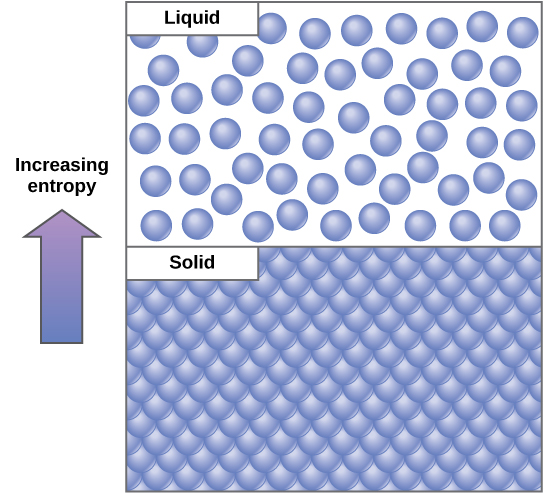Entropy: Entropy is a measure of randomness or disorder in a system. Gases have higher entropy than liquids, and liquids have higher entropy than solids.

Entropy changes also occur in chemical reactions. In an exergonic chemical reaction where energy is released, entropy increases because the final products have less energy inside them holding their chemical bonds together. That energy has been lost to the environment, usually in the form of heat.

All physical systems can be thought of in this way. Living things are highly ordered, requiring constant energy input to be maintained in a state of low entropy. As living systems take in energy-storing molecules and transform them through chemical reactions, they lose some amount of usable energy in the process because no reaction is completely efficient. They also produce waste and by-products that are not useful energy sources. This process increases the entropy of the system’s surroundings. Since all energy transfers result in the loss of some usable energy, the second law of thermodynamics states that every energy transfer or transformation increases the entropy of the universe. Even though living things are highly ordered and maintain a state of low entropy, the entropy of the universe in total is constantly increasing due to the loss of usable energy with each energy transfer that occurs. Essentially, living things are in a continuous uphill battle against this constant increase in universal entropy.

## Activation Energy

Activation energy is the energy required for a reaction to occur, and determines its rate.

### Learning Objectives

Discuss the concept of activation energy

### Key Takeaways

#### Key Points

• Reactions require an input of energy to initiate the reaction; this is called the activation energy (EA).
• Activation energy is the amount of energy required to reach the transition state.
• The source of the activation energy needed to push reactions forward is typically heat energy from the surroundings.
• For cellular reactions to occur fast enough over short time scales, their activation energies are lowered by molecules called catalysts.
• Enzymes are catalysts.

#### Key Terms

• activation energy: The minimum energy required for a reaction to occur.
• catalysis: The increase in the rate of a chemical reaction by lowering its activation energy.
• transition state: An intermediate state during a chemical reaction that has a higher energy than the reactants or the products.

Many chemical reactions, and almost all biochemical reactions do not occur spontaneously and must have an initial input of energy (called the activation energy) to get started. Activation energy must be considered when analyzing both endergonic and exergonic reactions. Exergonic reactions have a net release of energy, but they still require a small amount of energy input before they can proceed with their energy-releasing steps. This small amount of energy input necessary for all chemical reactions to occur is called the activation energy (or free energy of activation) and is abbreviated EA.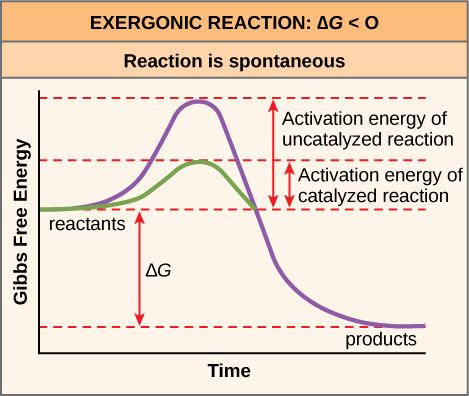Activation energy: Activation energy is the energy required for a reaction to proceed; it is lower if the reaction is catalyzed. The horizontal axis of this diagram describes the sequence of events in time.

### Activation Energy in Chemical Reactions

Why would an energy-releasing, negative ∆G reaction actually require some energy to proceed? The reason lies in the steps that take place during a chemical reaction. During chemical reactions, certain chemical bonds are broken and new ones are formed. For example, when a glucose molecule is broken down, bonds between the carbon atoms of the molecule are broken. Since these are energy-storing bonds, they release energy when broken. However, to get them into a state that allows the bonds to break, the molecule must be somewhat contorted. A small energy input is required to achieve this contorted state, which is called the transition state: it is a high-energy, unstable state. For this reason, reactant molecules don’t last long in their transition state, but very quickly proceed to the next steps of the chemical reaction.

Cells will at times couple an exergonic reaction $(\Delta \text{G}<0)$ with endergonic reactions $(\Delta \text{G}>0)$, allowing them to proceed. This spontaneous shift from one reaction to another is called energy coupling. The free energy released from the exergonic reaction is absorbed by the
endergonic reaction. One example of energy coupling using ATP involves a transmembrane ion pump that is extremely important for cellular function.

### Free Energy Diagrams

Free energy diagrams illustrate the energy profiles for a given reaction. Whether the reaction is exergonic (ΔG<0) or endergonic (ΔG>0) determines whether the products in the diagram will exist at a lower or higher energy state than the reactants. However, the measure of the activation energy is independent of the reaction’s ΔG. In other words, at a given temperature, the activation energy depends on the nature of the chemical transformation that takes place, but not on the relative energy state of the reactants and products.

Although the image above discusses the concept of activation energy within the context of the exergonic forward reaction, the same principles apply to the reverse reaction, which must be endergonic. Notice that the activation energy for the reverse reaction is larger than for the forward reaction.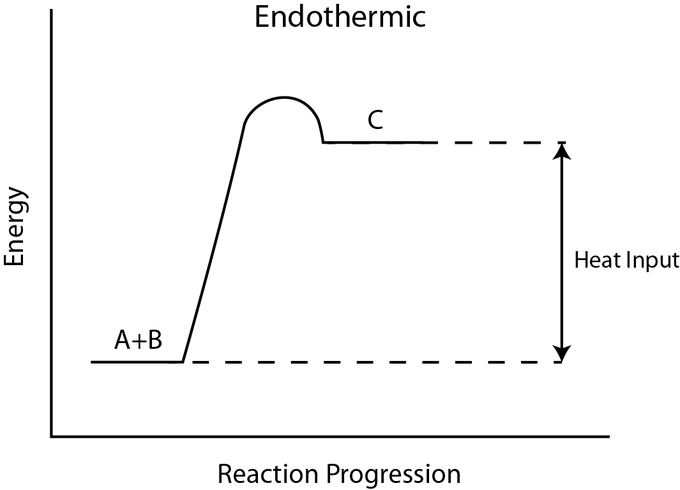Activation energy in an endergonic reaction: In this endergonic reaction, activation energy is still required to transform the reactants A + B into the product C. This figure implies that the activation energy is in the form of heat energy.

### Heat Energy

The source of the activation energy needed to push reactions forward is typically heat energy from the surroundings. Heat energy (the total bond energy of reactants or products in a chemical reaction) speeds up the motion of molecules, increasing the frequency and force with which they collide. It also moves atoms and bonds within the molecule slightly, helping them reach their transition state. For this reason, heating up a system will cause chemical reactants within that system to react more frequently. Increasing the pressure on a system has the same effect. Once reactants have absorbed enough heat energy from their surroundings to reach the transition state, the reaction will proceed.

The activation energy of a particular reaction determines the rate at which it will proceed. The higher the activation energy, the slower the chemical reaction will be. The example of iron rusting illustrates an inherently slow reaction. This reaction occurs slowly over time because of its high EA. Additionally, the burning of many fuels, which is strongly exergonic, will take place at a negligible rate unless their activation energy is overcome by sufficient heat from a spark. Once they begin to burn, however, the chemical reactions release enough heat to continue the burning process, supplying the activation energy for surrounding fuel molecules.

Like these reactions outside of cells, the activation energy for most cellular reactions is too high for heat energy to overcome at efficient rates. In other words, in order for important cellular reactions to occur at significant rates (number of reactions per unit time), their activation energies must be lowered; this is referred to as catalysis. This is a very good thing as far as living cells are concerned. Important macromolecules, such as proteins, DNA, and RNA, store considerable energy, and their breakdown is exergonic. If cellular temperatures alone provided enough heat energy for these exergonic reactions to overcome their activation barriers, the essential components of a cell would disintegrate.

### The Arrhenius Equation

The Arrhenius equations relates the rate of a chemical reaction to the magnitude of the activation energy:

$\text{k}=\text{A}\text{e}^{\text{E}_\text{a}/\text{RT}}$

where

• k is the reaction rate coefficient or constant
• A is the frequency factor of the reaction. It is determined experimentally.
• R is the Universal Gas constant
• T is the temperature in Kelvin